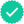#### Related questions#### Concept explainers

Question

An object has a position given by r = [(2.5 m/s^3)t^3 +(3.0 m/s)t]i +[3.0 m -(2.0 m/s^2)t^2]j where all quantities are in SI units. Calculate the magnitude of the acceleration of the object at time t = 2.00 s

Expert Solution